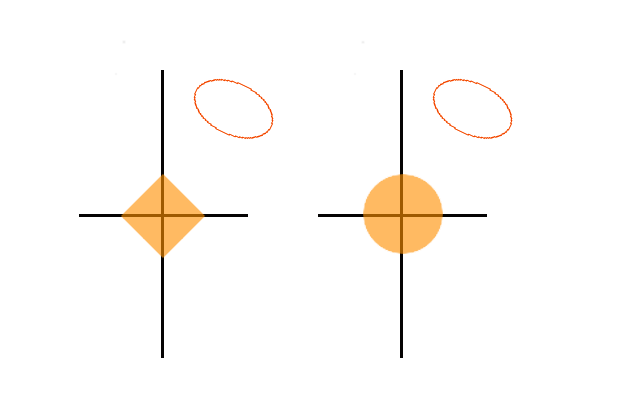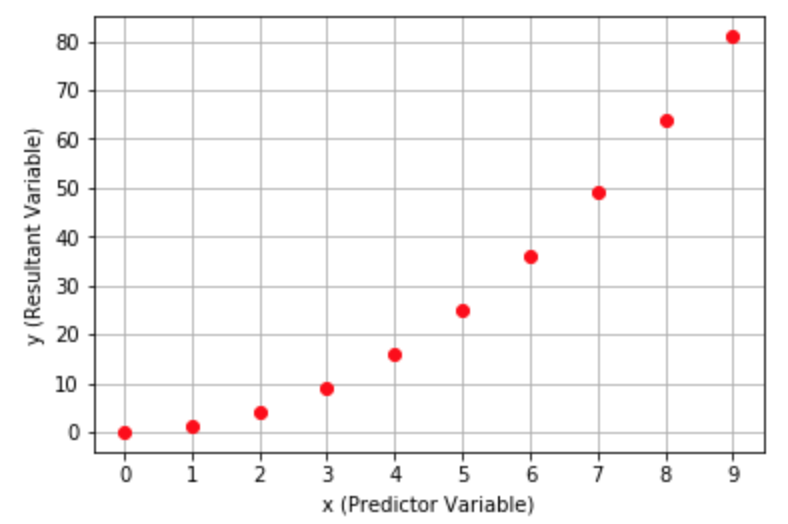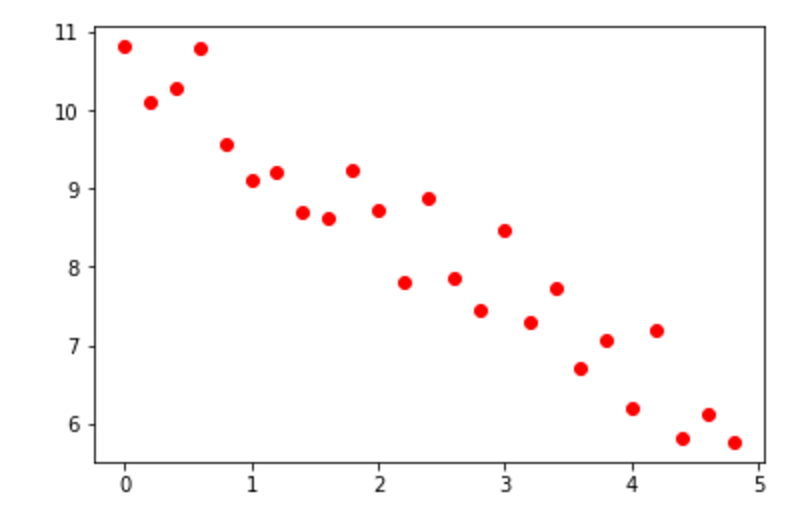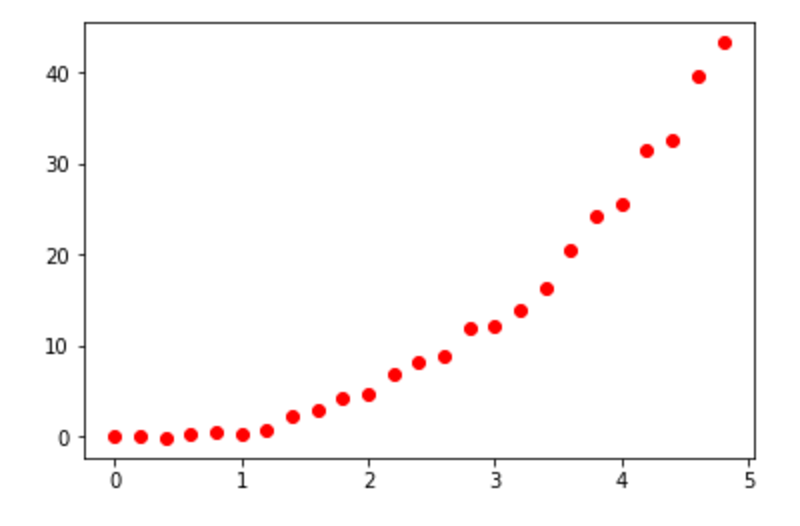### Machine Learning

Least squares linear regression is probably the most well-known type of regression, but there are many other variants which can minimize the problems associated with it.

A common one is known as ridge regression. This method is very similar to least squares regression but modifies the error function slightly.

Previously, we used the sum of square errors of the regression line as a measure of error, but in ridge regression we seek to minimize the squared values of coefficients as well. This gives the error function $\small \text{Error} = \sum_{i=1}^{n} (y_i - m_1x_{1i} - m_2x_{2i} - … - m_px_{pi} - b)^2 + \lambda \sum_{i=1}^{p} (m_i^2).$ Here, the value of lambda changes how aggressively coefficients are dampened. Notice that this error function does not penalize the size of the $y$-intercept.

# Alternatives to Linear Regression

A close relative to ridge regression is simply known as “lasso.” This also penalizes the size of coefficients in the error function, but does so based on their linear size instead of their squared size. Therefore, error is given by $\small \text{Error} = \sum_{i=1}^{n} (y_i - m_1x_{1i} - m_2x_{2i} - … - m_px_{pi} - b)^2 + \lambda \sum_{i=1}^{p} \vert m_i \vert.$

Below, we use a coding environment to import a LASSO class from the sklearn library and compared its results with those of normal linear regression. Press run to see the the results of each model plotted.

from sklearn import linear_model
import matplotlib.pyplot as plt
import numpy as np

#Generate artificial data for regression lines.
x = np.random.normal(0, 10, (20, 1))
y = 4*x+10+np.random.normal(0, 5, (20, 1))

regr = linear_model.LinearRegression()
LASSO = linear_model.Lasso(alpha=50) #50 is a large value of alpha, and is chosen for demonstration purposes.
plot_x = np.transpose(np.array([list(range(-20, 20))]))
regr.fit(x, y)
LASSO.fit(x, y)
plt.plot(plot_x, regr.predict(plot_x))
plt.plot(plot_x, LASSO.predict(plot_x))
plt.legend(["Linear Regression", "LASSO"])
plt.plot(x, y, "ro")
plt.axis([-15, 15, -70, 70])
plt.savefig("Plots.png", format="png")
Python 3
You need to be connected to run code

# Alternatives to Linear Regression

It is not at all obvious why lasso would have behavior significantly differing from ridge regression, but there is an interesting geometric reason for the differences. However, to demonstrate this, we must first change the way we view both techniques.

In ridge regression, it turns out that for any values of $\lambda$ we pick, it’s possible to find a value for $\lambda_2$ such that minimizing $\sum_{i=1}^{n} (y_i - m_1x_{1i} - m_2x_{2i} - … - m_px_{pi} - b)^2 + \lambda \sum_{i=1}^{p} \big(m_i^2\big)$ is equivalent to minimizing the SSE when $\sum_{i=1}^{p} \big(m_i^2\big) \leq \lambda_2.$

(This can be shown using the method of Lagrange multipliers)

Similarly, for any value of $\lambda$ there is some value of $\lambda_2$ such that using lasso is equivalent to minimizing the SSE when $\sum_{i=1}^{p} \vert m_i \vert \leq \lambda_2.$ A useful way to view the SSE when there are two predictor variables is shown in the pictures below. Here, the $x$- and $y$-axes represent the values of coefficients in a best-fit plane, and the ellipses show all pairs of coefficients which produce a certain value of the SSE for a data set. As the SSE increases, the ellipses get larger.Also in the pictures are two areas. The diamond represents the coefficient values allowed by lasso. The disk represents the possible coefficient values in ridge regression. Viewing these pictures, which form of linear regression will most likely lead to coefficients of zero?

# Alternatives to Linear Regression

Lasso generally behaves very similarly to ridge regression but, as we saw in the previous question, there is one crucial difference. It is capable of reducing the weights of predictor variables to zero. This is useful if one wants to cull predictor variables, among other things. Usually, this is done when there are many predictor variables and using too many in the model will cause overfitting or make it overcomplicated.

# Alternatives to Linear Regression

A group of scientists wants to analyze bacterial growth in Petri dishes. They have done a dozen tests, and each time they have recorded every single detail of the environment. The pH levels of the dishes, sugar content of the food, and even the light levels in the room have been recorded. A total of fourteen variables have been taken into account.

In a classic example of overzealous testing, a rogue scientist has added another variable to the mix, his average mood on a scale from zero to ten. When a best-fit equation is generated with this variable included, how will the SSE most likely change? How will the average error on new data change?

# Alternatives to Linear Regression

The previous question is a good example of a case where ridge regression or lasso would be very useful. Each of these techniques will penalize a best-fit line for having large coefficients, so they are likely to produce equations that make minimal use of predictor variables that have little sway over the result. Because the equation has a limited “budget,” it can only afford to give large weights to variables which are important.

In the case shown previously, this means that something with as little predictive power as the scientist’s mood the day of the test will be largely ignored. In fact, if lasso is used, the variable will probably be ignored entirely.

# Alternatives to Linear Regression

Of course, linear regression is just one of many techniques. A non-linear method with comparable simplicity is known as K-nearest neighbors regression.

To use K-nearest neighbors regression, or KNN regression for short, we must start with a data set. As with linear regression, the dataset must take of form of pairs of predictor variables $\vec{x}_i$ with resultant variables $y_i$. The goal is to use this dataset to predict the value of a resultant variable $y$ from a vector of predictor variables $\vec{x}$.

To make a prediction for $\vec{x}$, we plot each $\vec{x}_i$ in our dataset, ignoring the resultants, and pick out the $k$ points geometrically closest to $\vec{x}$. The estimate KNN regression provides is simply the average of the resultant values for these points.

One useful property of KNN regression is that it makes very few assumptions about the data sets it builds on. Unlike linear regression, which assumes linear relationships, KNN regression can accommodate nearly anything.

Additionally, by adjusting the value of $k,$ we can change the flexibility of KNN regression. If we want to account for even the smallest trends in our data set, we can pick a very small $k$-value. On the other hand, larger values of $k$ will eliminate smaller deviations in favor of larger trends.

# Alternatives to Linear Regression

Let's try applying KNN regression to a simple example. In the image below, we've plotted a dataset of ten points, where the predictor variable is given by the x-axis and the resultant variable is given by the y-axis. In this case, $y = x^2$ for all points in the dataset.Now, suppose that we have a new point for which $x = 3.5$ and we want to predict its value with KNN Regression. If we use $2$ as our value for K, what will our estimate be?

Hint: In KNN regression we pick out the K points geometrically closest to $\vec{x}$ and average their resultant values.

# Alternatives to Linear Regression

Below, we have three data sets—A, B, C—represented by either tables or scatter plots. We can analyze one with K-nearest neighbor regression, one with lasso, and one with normal linear regression. Which pairings will give the best results?

Data Set A: $\begin{array}{c|c|c|c|c} x_1 & x_2 & x_3 & x_4 & x_5 & x_6 & y \\ \hline 5 & 8 & 97 & 2 & 0 & 8 & 3 \\ \hline 2 & 7 & 0 & 2 & 1 & 7 & 4\\ \hline 2 & 6 & 4 & 12 & 6 & 3 & 14 \\ \hline 15 & 6 & -20 & 5 & 2 & 2 & 6 \\ \hline 4 & 8 & 2 & 6 & 0 & 3 & 5\\ \end{array}$

Data Set B:Data Set C:# Alternatives to Linear Regression

×